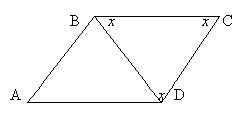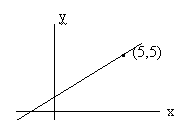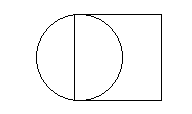## GMAT - Problem Solving - Test 5

#### 1. Sheila works 8 hours per day on Monday, Wednesday and Friday, and 6 hours per day on Tuesday and Thursday. She does not work on Saturday and Sunday. She earns \$324 per week. How much does she earn in dollars per hour?

A. 11
B. 10
C. 9
D. 8
E. 7A. 12
B. 9√3
C. 9
D. 8
E. 3√3

A. 2
B. 3
C. 4
D. 5
E. 6

#### I n is odd II n + 1 cannot be a prime number III (n + 2) divided by 7 has remainder 2

A. none
B. I only
C. I and II only
D. II and III only
E. I, II and III

#### 5. A solid cube of side 6 is first painted pink and then cut into smaller cubes of side 2. How many of the smaller cubes have paint on exactly 2 sides?

A. 30
B. 24
C. 12
D. 8
E. 6#### 6. The slope of the line passing through the point (5,5) is 5/6. All of the following points could be on the line except

A. (2.5, 2)
B. (11, 10)
C. (8, 7.5)
D. (-1, 0)
E. (-7, -5)A. 2a²
B. 4a
C. 4a²
D. 16a²
E. 64a²

A. 2
B. 4
C. 6
D. 8
E. 10

A. 60bm/c
B. bm/60c
C. bc/60m
D. 60b/cm
E. b/60cm

#### 10. Paint needs to be thinned to a ratio of 2 parts paint to 1.5 parts water. The painter has by mistake added water so that he has 6 litres of paint which is half water and half paint. What must he add to make the proportions of the mixture correct?

A. 1 litre paint
B. 1 litre water
C. ½ litre water and one litre paint
D. ½ litre paint and one litre water
E. ½ litre paint